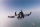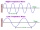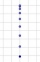# Car crash

On the road, with a maximum permitted speed of 60 km/h, there was a car crash. From the length of the vehicle's braking distance, which was 40 m, the police investigated whether the driver did not exceed that speed. What is the conclusion of the police, assuming a decelerated motion of the vehicle with a deceleration of 5 m/s2

Result

s =  27.778 m

#### Solution:Leave us a comment of example and its solution (i.e. if it is still somewhat unclear...):

Showing 0 comments:Be the first to comment!#### To solve this example are needed these knowledge from mathematics:

Do you want to convert length units?

## Next similar examples:

1. GravitationFrom the top of the 80m high tower, the body is thrown horizontally with an initial speed of 15 m/s. At what time and at what distance from the foot of the tower does the body hit the horizontal surface of the Earth? (use g = 10 ms-2)
2. Traffic collisionWhen investigating a traffic accident, it was found that the driver stopped the vehicle immediately after the accident by constant braking on a 150 m track in 15 seconds. Do you approve that the driver exceeded the permitted speed (50 km/h) in the village.
3. BomberThe aircraft flies at an altitude of 4100 m above the ground at speed 777 km/h. At what horizontal distance from the point B should be release any body from the aircraft body to fall into point B? (g = 9.81 m/s2)
4. Aircraft nose downHow long will fall airliner from a height of 10000 m at speed 1,000 km/h?
5. CollisionThe two bodies, whose initial distance is 240 m, move evenly against each other consistently. The first body has an initial velocity of 4 m/s and an acceleration of 3 m/s2, the second body has an initial speed of 6 m/s and an acceleration of 2 m/s2. Find
6. G forcesCalculate deceleration of car (as multiple of gravitational acceleration g = 9.81 m/s2) which occurs when a car in a frontal collision slows down uniformly from a speed 111 km/h to 0 km/h in 1.2 meters trajectory.
7. Free fallHow long does the stone fall freely into a depth of 80m? What speed will it hit the bottom of the abyss?
8. AbyssStone was pushed into the abyss: 2 seconds after we heard hitting the bottom. How deep is the abyss (neglecting air resistance)? (gravitational acceleration g = 9.81 m/s2 and the speed of sound in air v = 343 m/s)
9. Wave parametersCalculate the speed of a wave if the frequency is 336 Hz and the wavelength is 10 m.
10. Free fallThe free fall body has gone 10m in the last 0.5s. Find the body speed at the moment of impact.
11. WavelengthCalculate the wavelength of the tone frequency 11 kHz if the sound travels at speeds of 343 m/s.
12. Train 2The train slowed down from 90 km/h to 72 km/h in 5 seconds. How long track travel?
13. WalkersWalker, which makes 120 steps per minute, make distance from point A to point B for 55 minutes. The length of his step is 75cm. For how long does this distance go boy who will do 110 steps 60 cm long in a minute?
14. Theorem proveWe want to prove the sentense: If the natural number n is divisible by six, then n is divisible by three. From what assumption we started?
15. OverloadWhat overload in g (g-force) has passed the pilot if he accelerated from 0 to 600 km/h in 3 seconds?
16. Rocket startThe body launched vertically up returns to the start site in 6 seconds. What height did it have?
17. Pre účinnosťPre účinnosť bŕzd osobného vozidla je predpísané, že automobil pohybujúci sa po vodorovnej vozovke rýchlosťou 40km. H musí zastaviť na dráhe 15,4 m. Aké veľké je pritom spomalenie automobilu?